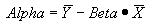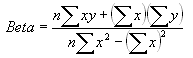Alpha Calculation

Alpha is a measure of an investment's performance relative to a benchmark, beyond what would be predicted by beta.  This benchmark is commonly the S&P 500, but Fund Manager allows you to assign any investment as the benchmark.  See the Benchmark Dialog.  A benchmark must be assigned before alpha calculations can be made.

Alpha is expressed as an annualized return percentage.  This reflects the additional (if greater than 0) excess performance over the benchmark, that would be predicted by beta alone.

#### Calculation Details:

When calculating the alpha of an investment the simple monthly returns over the specified comparison period are calculated.  The simple monthly return is:

Return = (End_price + Dist_per_share - Start_price) / Start_price

"Excess returns" are then calculated based off these returns and the "risk-free" return specified in the Benchmark Dialog

Excess Return = Return - Risk_Free_Return

The monthly excess returns for both the investment and benchmark are calculated.  If the comparison period is 5 years, there are 60 monthly excess returns for each the investment and the benchmark.  To calculate the alpha, these 60 data points are plotted with the benchmark excess returns along the X axis, and the investment excess returns along the Y axis. The Y-axis intercept of a best-fit line through these data points is the Alpha.where Beta is:where 'n' is the number of data points (60 in this example), and 'x' are the benchmark returns, and 'y' are the investment returns.  When calculating returns your current interpolation range preferences are used.  If sufficient pricing data is not available the alpha will not be reported.

The alpha for a portfolio, asset type, goal, or investment type is determined by calculating excess returns from a weighted average of the investments in that group.  The weighting is based on the ending value.

Fund Manager can output the data points used in this calculation to a log file.  By default the logging feature is turned off.  To turn it on, create a new registry string value at:

HKEY_CURRENT_USER\Software\Beiley Software\Fund Manager\CurrentVersion\techanal

named "benchmarklog" and set it to a value of "1".  The log file is named "stats_log.txt", and will be located in your user's AppData\Roaming\Fund Manager folder (usually a hidden folder).  This log file is deleted upon exiting Fund Manager.